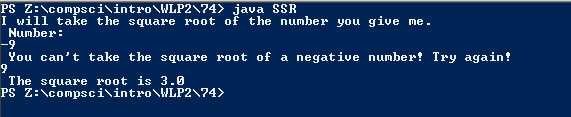# Assignment #74 Safe Square Root

## Code

```    ///Name: Morgan Kaplan
///Period: 5
///Project Name: Safe Square Root
///File Name: SSR.java

import java.util.Scanner;
public class SSR
{
public static void main( String[] args)
{
Scanner momo= new Scanner(System.in);

int tries=1;

System.out.println( "I will take the square root of the number you give me.");
System.out.println( " Number: " );
int Number=momo.nextInt();

while ( Number < 0 )
{

System.out.println(" You can't take the square root of a negative number! Try again! ");
Number=momo.nextInt();
tries++;
}
double done= Math.sqrt(Number);

System.out.println( " The square root is " + done + " ");

}
}
```

### Picture of Output